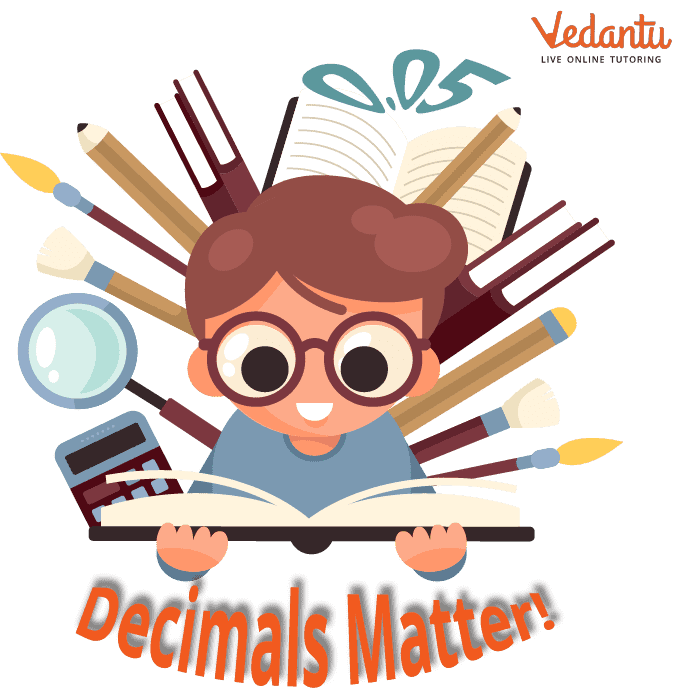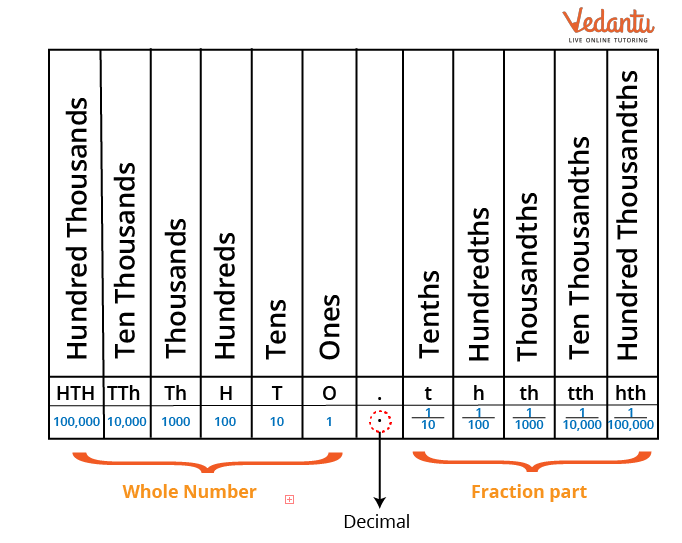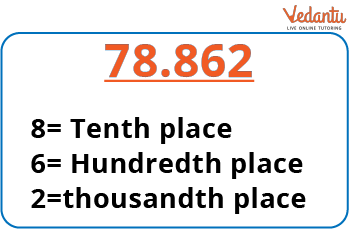Courses
Courses for Kids
Free study material
Free LIVE classes
More

# One Decimal PlaceLIVE
Join Vedantu’s FREE Mastercalss

## Introduction

There are two components to a decimal: a whole and a fraction. When a whole is divided into smaller parts, decimals are created. Every part of a decimal number uses the same decimal place value system as the whole number. The decimal number's fractional components are located after the decimal point.Decimal

## Decimal Place Value Chart:

Ones are the first place value before the decimal point, followed by tens, hundreds, and so forth. Whereas the place values after the decimal point begin with tenths and go through hundredths, thousandths, and so forth. The place value that comes after the decimal represents the fractional part of the number.Chart on Decimal Place Value

## How to Round to One Decimal Place:

To the right of the decimal point are different names for the place values. The number at the hundredth point place will affect whether you round up or down when you round to the first decimal place or the nearest tenth. “Roundup” to the next whole number if the hundredth digit is a number between 5 and 9. If the number lies between 0 and 4, you "round down" by maintaining the same tenth place.

Let us take an example of the number 78.86 to get a better understanding.78.862

Here, the thousandth digit is 2, less than 5; hence, the next hundredth value will round down and maintain the same hundredth-place value. The number is: 78.86.

Now, the hundredth digit is 6, between 5 and 9; we will round up the tenth decimal point to 9. The resulting number from rounding to the nearest tenth will be 9. Now, the number is: 78.9

Therefore, the obtained result will be 78.9

There are also some correct-to-one-decimal place calculators to carry out the process successfully.

## Examples of correcting to One Decimal Place:

Let us look at a few one-decimal-place examples:

Example 1: Round to the nearest tenths: 14.235

Solution: From the given question, the number is 14.235.

Here, the thousandth digit is 5, between 5 and 9; we will round up the hundredth decimal point to 9. The number from rounding to the nearest hundredth place value will be 4. The number is:

14.24

The hundredth digit is 4, less than 5; hence, the next tenth value will round down and maintain the same tenth-place value. The number is:

14.2

Therefore, the obtained result will be 14.2

Example 2: Round to the nearest tenths: 6.31

Solution: From the given question, the number is 6.31

Here, the hundredth digit is 1, less than 5; hence, the next tenth value will round down and maintain the same tenth-place value. The number is:

6.3

Therefore, the obtained result will be 6.3.

Example 3: Round to the nearest tenths: 0.2856

Solution: From the given question, the number is 0.2856.

Here, the ten-thousandth digit is 6, between 5 and 9; we will round up the thousandth decimal point to 9. The number from rounding to the nearest thousandth place value will be 6. The number is:

0.286

The thousandth digit is 6, between 5 and 9; we will round up the hundredth decimal point to 9. The number from rounding to the nearest hundredth place value will be 9. The number is:

0.29

At last, the hundredth digit is 9, between 5 and 9; we will round up the tenth decimal point to 9. The resulting number from rounding to the nearest tenth-place value will be 3. The number is:

0.3

Therefore, the obtained result will be 0.3.

## Conclusion:

To round off a number to one decimal place, the names of each decimal place to the right of the decimal point must be known. The whole number is read first, just like any other whole number, followed by the decimal point, which is read as a "point," and then each digit of the other part. The decimal dot should be viewed as "and," followed by reading the complete fractional component while keeping in mind the place value of the final digit. This is a more formal approach to reading decimals.

Last updated date: 22nd Sep 2023
Total views: 78.6k
Views today: 0.78k

## FAQs on One Decimal Place

1. Why use one decimal place when rounding?

Because some numbers have many decimal places, it's crucial to know how to round to one. Therefore, we must round down to the next decimal point to maintain clarity.

2. What are the types of decimals?

The types of decimals are: like and unlike decimals.

• Like Decimals: If there are the same number of digits following the decimal point in two decimal numbers, they are said to be "like" decimals.

• Unlike Decimals: If there are different amounts of digits following the decimal point in two decimal numbers, they are said to be "unlike" decimals.

3. What does decimal mean?

The word Decimus, which means tenth in Latin, is derived from the base word Decem, or 10. As a result, the decimal system, often known as a base-10 system, has 10 as its fundamental unit. A number expressed using the decimal method is also called a "decimal."